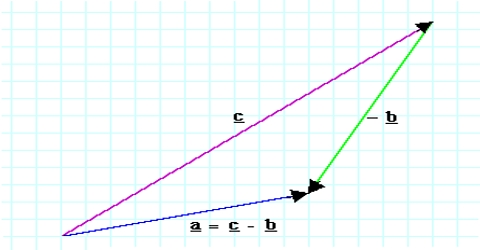# Law of Components of Vector Subtraction

Law of components of vector subtraction:

In any coordinate system a vector quantity can be resolved into components. Determine the resultant vector by resolving each vector quantity in its components and then adding by algebraic rule.

If OX, OY, OZ are three axes in three dimensional coordinate system, coordinates of the position vector Rx is (Ax, Ay, Az) and its components along X, Yand Z axes are Axî, Ayĵ and Azƙ; then

Ā =  Axî + Ayĵ + Azƙ

Similarly, the vector B can be written along X, Y and Z axes in terms of components as,

B = Bxî + Byĵ + Bzƙ

Modulus of the resultant: Modulus at the resultant of vector subtraction of Ā and B is,

Addition of these two vectors is: R = A – BThe two vectors A and B can be written in resolved components as;

Ā = Axî + Ayĵ + Azƙ and B = Bxî + Byĵ + Bzƙ. Subtraction of these two vectors will be; A – B.

So, Modules of the resultant is,

|C| = |A – B| = √(Cx2 + Cy2 + Cz2)

= √[(Ax-Bx)2 + (Ay-By)2 + (Az-Bz)2]

In two dimensional cases, quantities associated with the components î and ĵ will remain.